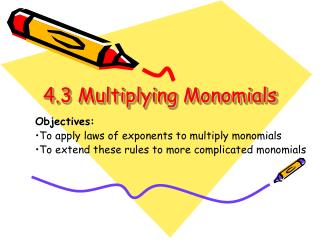# 4.3 Multiplying Monomials - PowerPoint PPT PresentationDownload Presentation4.3 Multiplying Monomials

4.3 Multiplying MonomialsDownload Presentation## 4.3 Multiplying Monomials

- - - - - - - - - - - - - - - - - - - - - - - - - - - E N D - - - - - - - - - - - - - - - - - - - - - - - - - - -
##### Presentation Transcript

1. 4.3 Multiplying Monomials Objectives: To apply laws of exponents to multiply monomials To extend these rules to more complicated monomials

2. Rules for Combining Like Terms Recall that there are two requirements for being able to COMBINE LIKE TERMS • Same variables • Same exponents

3. Rules for Combining Like Terms Alien Code #1: To ride in the same spaceship, you must have • Same BODY • Same NUMBER OF HEADS

4. Rules for Combining Like Terms Alien Code #1: To ride in the same spaceship, you must have • Same BODY • Same NUMBER OF HEADS Leave variables alone Add the coefficients

5. 3X2 + 1Y3 + 3Z4 + 2X2 + 1Y3 + 2X2 + 3Y3 + 1Z4 7X2 + 5Y3 + 4Z4 Leave variables alone Add the coefficients

6. Let’s try it with some more complicated monomials 3x2y3z4 + 5xy3z4 - 2x2y3z4 + 3xy3z4 1x2y3z4+ 8xy3z4 Leave variables alone Add the coefficients

7. Rules for Multiplying Monomials Let’s extend this now to apply to when we multiply monomials • Multiply coefficients together • For exponents with same base (variable), add the exponents together

8. Rules for Multiplying Monomials Alien Code #2: If aliens bump into each other, you have to • MULTIPLY the coefficients • Squish them into the same BODY • ADD the NUMBER OF HEADS

9. Rules for Multiplying Monomials Let’s extend this now to apply to when we multiply monomials • Multiply coefficients together • For exponents with same base (variable), add the exponents together X2 TIMESX2 X*X TIMES X*X X*X*X*X X4

10. 34 3X2 TIMES4X2 (3)(4) 12X4

11. Examples Simplify • (5x2)(-2x2) • (-3x3)(3x4) • (2y3)(3y4)

12. Multiplying Monomials (-3x3y2z5)(3x2y3z) -9x • MULTIPLY the coefficients • Squish them into the same BODY

13. Multiplying Monomials (-3x3y2z5)(3x2y3z) -9x5 • MULTIPLY the coefficients • Squish them into the same BODY • ADD the NUMBER OF HEADS

14. Multiplying Monomials (-3x3y2z5)(3x2y3z) -9x5y • MULTIPLY the coefficients • Squish them into the same BODY

15. Multiplying Monomials (-3x3y2z5)(3x2y3z) -9x5y5 • MULTIPLY the coefficients • Squish them into the same BODY • ADD the NUMBER OF HEADS

16. Multiplying Monomials (-3x3y2z5)(3x2y3z) -9x5y5z • MULTIPLY the coefficients • Squish them into the same BODY

17. Multiplying Monomials (-3x3y2z5)(3x2y3z1) -9x5y5z6 • MULTIPLY the coefficients • Squish them into the same BODY • ADD the NUMBER OF HEADS

18. Examples Simplify • (5x2y3)(3x2y4) • (-3x3y2z5)(3x2y3z) • (2x2y3z3)(3x2y4)